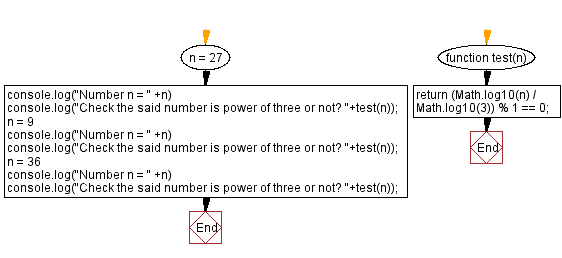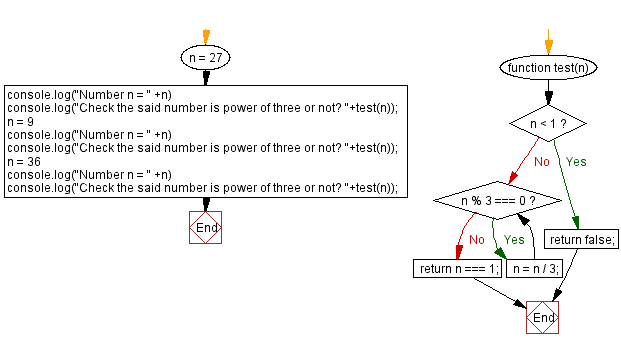# JavaScript: Power of three

## JavaScript Math: Exercise-107 with Solution

Write a JavaScript program to check whether a given integer is a power of three or not.

In mathematics, a power of three is a number of the form 3n where n is an integer – that is, the result of exponentiation with number three as the base and integer n as the exponent.

Test Data:
(27) -> true
(9) -> true
(36) -> false

Sample Solution:

HTML Code:

``````<!DOCTYPE html>
<html>
<meta charset="utf-8">
<title>JavaScript program to Power of three or not</title>
<body>

</body>
</html>
```
```

Solution-1

JavaScript Code:

``````function test(n) {
return (Math.log10(n) / Math.log10(3)) % 1 == 0;
}

n = 27
console.log("Number n = " +n)
console.log("Check the said number is power of three or not? "+test(n));
n = 9
console.log("Number n = " +n)
console.log("Check the said number is power of three or not? "+test(n));
n = 36
console.log("Number n = " +n)
console.log("Check the said number is power of three or not? "+test(n));
```
```

Sample Output:

```Number n = 27
Check the said number is power of three or not? true
Number n = 9
Check the said number is power of three or not? true
Number n = 36
Check the said number is power of three or not? false
```

Flowchart:Live Demo:

See the Pen javascript-math-exercise-107 by w3resource (@w3resource) on CodePen.

Solution-2

JavaScript Code:

``````function test(n) {
if (n < 1) {
return false;
}
while (n % 3 === 0) {
n = n / 3;
}
return n === 1;
}
n = 27
console.log("Number n = " +n)
console.log("Check the said number is power of three or not? "+test(n));
n = 9
console.log("Number n = " +n)
console.log("Check the said number is power of three or not? "+test(n));
n = 36
console.log("Number n = " +n)
console.log("Check the said number is power of three or not? "+test(n));
```
```

Sample Output:

```Number n = 27
Check the said number is power of three or not? true
Number n = 9
Check the said number is power of three or not? true
Number n = 36
Check the said number is power of three or not? false
```

Flowchart:Live Demo:

See the Pen javascript-math-exercise-107-1 by w3resource (@w3resource) on CodePen.

Improve this sample solution and post your code through Disqus

What is the difficulty level of this exercise?

Test your Programming skills with w3resource's quiz.

﻿